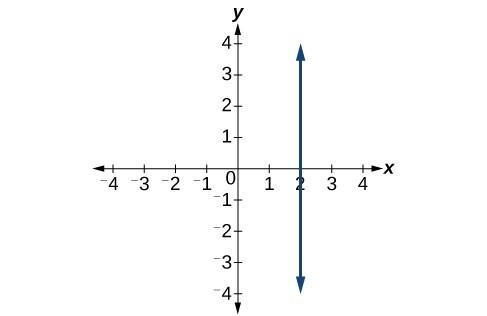## Section 1.4: Linear Functions and Their Graphs

### Learning Outcomes

• Identify and Interpret the Slope and Vertical Intercept of a Line
• Calculate and interpret the slope of a line.
• Determine the equation of a linear function.
• Graph linear functions.
• Write the equation for a linear function from the graph of a line.

Introduction to Linear Functions

A bamboo forest in China (credit: “JFXie”/Flickr)Imagine placing a plant in the ground one day and finding that it has doubled its height just a few days later. Although it may seem incredible, this can happen with certain types of bamboo species. These members of the grass family are the fastest-growing plants in the world. One species of bamboo has been observed to grow nearly 1.5 inches every hour.  In a twenty-four hour period, this bamboo plant grows about 36 inches, or an incredible 3 feet! A constant rate of change, such as the growth cycle of this bamboo plant, is a linear function.

Recall from Functions and Function Notation that a function is a relation that assigns to every element in the domain exactly one element in the range. Linear functions are a specific type of function that can be used to model many real-world applications, such as plant growth over time. In this chapter, we will explore linear functions, their graphs, and how to relate them to data.

Just as with the growth of a bamboo plant, there are many situations that involve constant change over time. Consider, for example, the first commercial maglev train in the world, the Shanghai MagLev Train. It carries passengers comfortably for a 30-kilometer trip from the airport to the subway station in only eight minutes.

Suppose a maglev train were to travel a long distance, and that the train maintains a constant speed of 83 meters per second for a period of time once it is 250 meters from the station. How can we analyze the train’s distance from the station as a function of time? In this section, we will investigate a kind of function that is useful for this purpose, and use it to investigate real-world situations such as the train’s distance from the station at a given point in time.

## Representing Linear Functions

The function describing the train’s motion is a linear function, which is defined as a function with a constant rate of change, that is, a polynomial of degree 1. There are several ways to represent a linear function, including word form, function notation, tabular form, and graphical form. We will describe the train’s motion as a function using each method.

### Representing a Linear Function in Word Form

Let’s begin by describing the linear function in words. For the train problem we just considered, the following word sentence may be used to describe the function relationship.

• The train’s distance from the station is a function of the time during which the train moves at a constant speed plus its original distance from the station when it began moving at constant speed.

The speed is the rate of change. Recall that a rate of change is a measure of how quickly the dependent variable changes with respect to the independent variable. The rate of change for this example is constant, which means that it is the same for each input value. As the time (input) increases by 1 second, the corresponding distance (output) increases by 83 meters. The train began moving at this constant speed at a distance of 250 meters from the station.

### Representing a Linear Function in Function Notation

Another approach to representing linear functions is by using function notation. One example of function notation is an equation written in the form known as the slope-intercept form of a line, where $x$ is the input value, $m$ is the rate of change, and $b$ is the initial value of the dependent variable.

\begin{align}&\text{Equation form} && y=mx+b \\ &\text{Function notation} && f(x)=mx+b\\& \end{align}

In the example of the train, we might use the notation $D\left(t\right)$ in which the total distance $D$
is a function of the time $t$. The rate, $m$, is 83 meters per second. The initial value of the dependent variable $b$ is the original distance from the station, 250 meters. We can write a generalized equation to represent the motion of the train.

$D(t)=83t+250$

### Representing a Linear Function in Tabular Form

A third method of representing a linear function is through the use of a table. The relationship between the distance from the station and the time is represented in the table in Figure 1. From the table, we can see that the distance changes by 83 meters for every 1 second increase in time.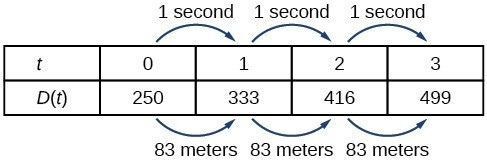Figure 1. Tabular representation of the function D showing selected input and output values

### Q & A

Can the input in the previous example be any real number?

No. The input represents time, so while nonnegative rational and irrational numbers are possible, negative real numbers are not possible for this example. The input consists of non-negative real numbers.

### Representing a Linear Function in Graphical Form

Another way to represent linear functions is visually, using a graph. We can use the function relationship from above, $D(t)=83t+250$, to draw a graph, represented in the graph in Figure 2. Notice the graph is a line. When we plot a linear function, the graph is always a line.

The rate of change, which is constant, determines the slant, or slope of the line. The point at which the input value is zero is the vertical intercept, or y-intercept, of the line. We can see from the graph that the y-intercept in the train example we just saw is $\left(0,250\right)$ and represents the distance of the train from the station when it began moving at a constant speed.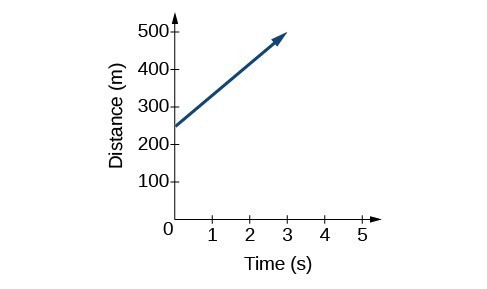Figure 2. The graph of $D(t)=83t+250$. Graphs of linear functions are lines because the rate of change is constant.

Notice that the graph of the train example is restricted, but this is not always the case. Consider the graph of the line $f(x)=2{x}_{}+1$. Ask yourself what numbers can be input to the function, that is, what is the domain of the function? The domain is comprised of all real numbers because any number may be doubled, and then have one added to the product.

### A General Note: Linear Function

A linear function is a function whose graph is a line. Linear functions can be written in the slope-intercept form of a line

$f(x)=mx+b$

where $b$ is the initial or starting value of the function (when input, $x=0$), and $m$ is the constant rate of change, or slope of the function. The y-intercept is at $\left(0,b\right)$.

### Example 1: Using a Linear Function to Find the Pressure on a Diver

The pressure, $P$, in pounds per square inch (PSI) on the diver in Figure 3 depends upon her depth below the water surface, $d$, in feet. This relationship may be modeled by the equation, $P(d)=0.434d+14.696$. Restate this function in words.Figure 3. (credit: Ilse Reijs and Jan-Noud Hutten)

## Determining Whether a Linear Function is Increasing, Decreasing, or Constant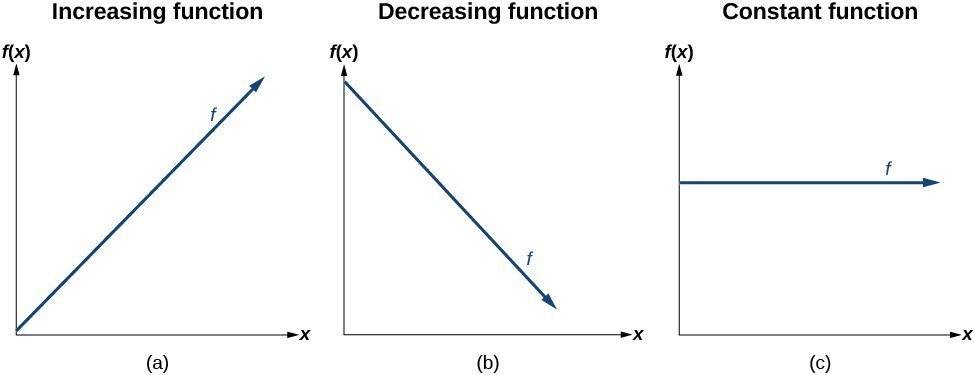Figure 4

The linear functions we used in the two previous examples increased over time, but not every linear function does. A linear function may be increasing, decreasing, or constant.

For an increasing function, as with the train example,

the output values increase as the input values increase.

The graph of an increasing function has a positive slope. A line with a positive slope slants upward from left to right as in (a).

For a decreasing function, the slope is negative.

The output values decrease as the input values increase.

A line with a negative slope slants downward from left to right as in (b). If the function is constant, the output values are the same for all input values so the slope is zero. A line with a slope of zero is horizontal as in (c).

### A General Note: Increasing and Decreasing Functions

The slope determines if the function is an increasing linear function, a decreasing linear function, or a constant function.

• $f(x)=mx+b\text{ is an increasing function if }m>0$.
• $f(x)=mx+b\text{ is an decreasing function if }m<0$.
• $f(x)=mx+b\text{ is a constant function if }m=0$.

### Example 2: Deciding whether a Function Is Increasing, Decreasing, or Constant

Some recent studies suggest that a teenager sends an average of 60 texts per day. For each of the following scenarios, find the linear function that describes the relationship between the input value and the output value. Then, determine whether the graph of the function is increasing, decreasing, or constant.

1. The total number of texts a teen sends is considered a function of time in days. The input is the number of days, and output is the total number of texts sent.
2. A teen has a limit of 500 texts per month in his or her data plan. The input is the number of days, and output is the total number of texts remaining for the month.
3. A teen has an unlimited number of texts in his or her data plan for a cost of 50 per month. The input is the number of days, and output is the total cost of texting each month. ## Calculating and Interpreting Slope In the examples we have seen so far, we have had the slope provided for us. However, we often need to calculate the slope given input and output values. Given two values for the input, ${x}_{1}$ and ${x}_{2}$, and two corresponding values for the output, ${y}_{1}$ and ${y}_{2}$ —which can be represented by a set of points, $\left({x}_{1}\text{, }{y}_{1}\right)$ and $\left({x}_{2}\text{, }{y}_{2}\right)$—we can calculate the slope $m$, as follows $m=\frac{\text{change in output (rise)}}{\text{change in input (run)}}=\frac{\Delta y}{\Delta x}=\frac{{y}_{2}-{y}_{1}}{{x}_{2}-{x}_{1}}$  where $\Delta y$ is the vertical displacement and $\Delta x$ is the horizontal displacement. Note in function notation two corresponding values for the output ${y}_{1}$ and ${y}_{2}$ for the function $f$, ${y}_{1}=f\left({x}_{1}\right)$ and ${y}_{2}=f\left({x}_{2}\right)$, so we could equivalently write $m=\frac{f\left({x}_{2}\right)-f\left({x}_{1}\right)}{{x}_{2}-{x}_{1}}$  The graph in Figure 5 indicates how the slope of the line between the points, $\left({x}_{1,}{y}_{1}\right)$ and $\left({x}_{2,}{y}_{2}\right)$, is calculated. Recall that the slope measures steepness. The greater the absolute value of the slope, the steeper the line is.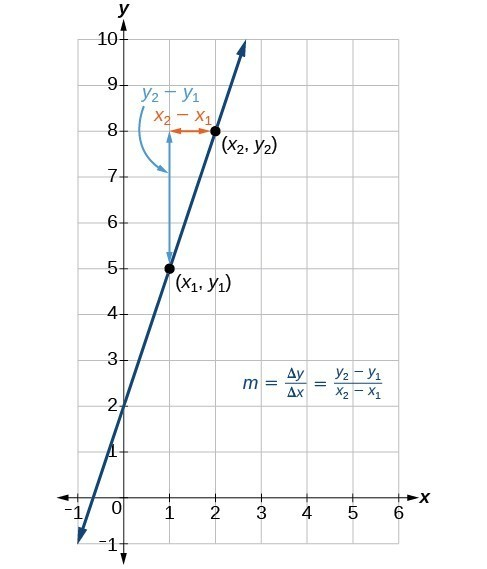Figure 5 The slope of a function is calculated by the change in $y$ divided by the change in $x$. It does not matter which coordinate is used as the $\left({x}_{2}\text{,}{y}_{2}\right)$ and which is the $\left({x}_{1}\text{,}{y}_{1}\right)$, as long as each calculation is started with the elements from the same coordinate pair. ### Q & A Are the units for slope always $\frac{\text{units for the output}}{\text{units for the input}}$ ? Yes. Think of the units as the change of output value for each unit of change in input value. An example of slope could be miles per hour or dollars per day. Notice the units appear as a ratio of units for the output per units for the input. ### A General Note: Calculate Slope The slope, or rate of change, of a function $m$ can be calculated according to the following: $m=\frac{\text{change in output (rise)}}{\text{change in input (run)}}=\frac{\Delta y}{\Delta x}=\frac{{y}_{2}-{y}_{1}}{{x}_{2}-{x}_{1}}$  where ${x}_{1}$ and ${x}_{2}$ are input values, ${y}_{1}$ and ${y}_{2}$ are output values. ### How To: Given two points from a linear function, calculate and interpret the slope. 1. Determine the units for output and input values. 2. Calculate the change of output values and change of input values. 3. Interpret the slope as the change in output values per unit of the input value. ### Example 3: Finding the Slope of a Linear Function If $f\left(x\right)$ is a linear function, and $\left(3,-2\right)$ and $\left(8,1\right)$ are points on the line, find the slope. Is this function increasing or decreasing? ### Try It If $f\left(x\right)$ is a linear function, and $\left(2,\text{ }3\right)$ and $\left(0,\text{ }4\right)$ are points on the line, find the slope. Is this function increasing or decreasing? ### Try It ### Example 4: Finding the Population Change from a Linear Function The population of a city increased from 23,400 to 27,800 between 2008 and 2012. Find the change of population per year if we assume the change was constant from 2008 to 2012. ### Try It The population of a small town increased from 1,442 to 1,868 between 2009 and 2012. Find the change of population per year if we assume the change was constant from 2009 to 2012. ### Try It ## Writing the Point-Slope Form of a Linear Equation Up until now, we have been using the slope-intercept form of a linear equation to describe linear functions. Here, we will learn another way to write a linear function, the point-slope form. $y-{y}_{1}=m\left(x-{x}_{1}\right)$  The point-slope form is derived from the slope formula. \begin{align}&{m}=\frac{y-{y}_{1}}{x-{x}_{1}} && \text{assuming }{ x }\ne {x}_{1} \\ &{ m }\left(x-{x}_{1}\right)=\frac{y-{y}_{1}}{x-{x}_{1}}\left(x-{x}_{1}\right) && \text{Multiply both sides by }\left(x-{x}_{1}\right)\\ &{ m }\left(x-{x}_{1}\right)=y-{y}_{1} && \text{Simplify} \\ &y-{y}_{1}={ m }\left(x-{x}_{1}\right) &&\text{Rearrange} \end{align}  Keep in mind that the slope-intercept form and the point-slope form can be used to describe the same function. We can move from one form to another using basic algebra. For example, suppose we are given an equation in point-slope form, $y - 4=-\frac{1}{2}\left(x - 6\right)$ . We can convert it to the slope-intercept form as shown. \begin{align}&y - 4=-\frac{1}{2}\left(x - 6\right) \\ &y - 4=-\frac{1}{2}x+3 && \text{Distribute the }-\frac{1}{2}. \\ &y=-\frac{1}{2}x+7 && \text{Add 4 to each side}. \end{align}  Therefore, the same line can be described in slope-intercept form as $y=-\frac{1}{2}x+7$. ### A General Note: Point-Slope Form of a Linear Equation The point-slope form of a linear equation takes the form $y-{y}_{1}=m\left(x-{x}_{1}\right)$ where $m$ is the slope, ${x}_{1 }$ and ${y}_{1}$ are the $x$ and $y$ coordinates of a specific point through which the line passes. ## Writing the Equation of a Line Using a Point and the Slope The point-slope form is particularly useful if we know one point and the slope of a line. Suppose, for example, we are told that a line has a slope of 2 and passes through the point $\left(4,1\right)$. We know that $m=2$ and that ${x}_{1}=4$ and ${y}_{1}=1$. We can substitute these values into the general point-slope equation. $\begin{gathered}y-{y}_{1}=m\left(x-{x}_{1}\right)\\ y - 1=2\left(x - 4\right)\end{gathered}$  If we wanted to then rewrite the equation in slope-intercept form, we apply algebraic techniques. \begin{align}&y - 1=2\left(x - 4\right) \\ &y - 1=2x - 8 && \text{Distribute the }2 \\ &y=2x - 7 && \text{Add 1 to each side} \end{align}  Both equations, $y - 1=2\left(x - 4\right)$ and $y=2x - 7$, describe the same line. See Figure 6.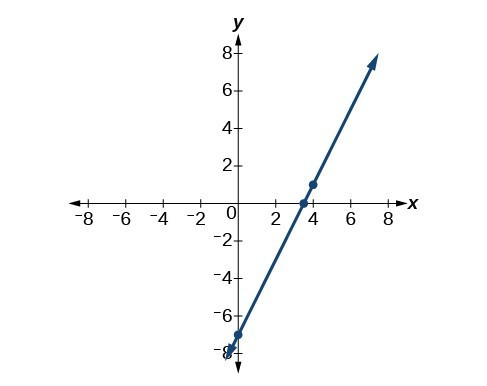Figure 6 ### Example 5: Writing Linear Equations Using a Point and the Slope Write the point-slope form of an equation of a line with a slope of 3 that passes through the point $\left(6,-1\right)$. Then rewrite it in the slope-intercept form. ### Try It Write the point-slope form of an equation of a line with a slope of –2 that passes through the point $\left(-2,\text{ }2\right)$. Then rewrite it in the slope-intercept form. ### Try It ## Writing the Equation of a Line Using Two Points The point-slope form of an equation is also useful if we know any two points through which a line passes. Suppose, for example, we know that a line passes through the points $\left(0,\text{ }1\right)$ and $\left(3,\text{ }2\right)$. We can use the coordinates of the two points to find the slope. \begin{align}{m}&=\frac{{y}_{2}-{y}_{1}}{{x}_{2}-{x}_{1}}\\ &=\frac{2 - 1}{3 - 0} \\ &=\frac{1}{3} \end{align}  Now we can use the slope we found and the coordinates of one of the points to find the equation for the line. Let use (0, 1) for our point. \begin{align}y-{y}_{1}&=m\left(x-{x}_{1}\right)\\ y - 1&=\frac{1}{3}\left(x - 0\right)\end{align}  As before, we can use algebra to rewrite the equation in the slope-intercept form. \begin{align}&y - 1=\frac{1}{3}\left(x - 0\right) \\ &y - 1=\frac{1}{3}x && \text{Distribute the }\frac{1}{3} \\ &y=\frac{1}{3}x+1 && \text{Add 1 to each side} \end{align}  Both equations describe the line shown in Figure 7.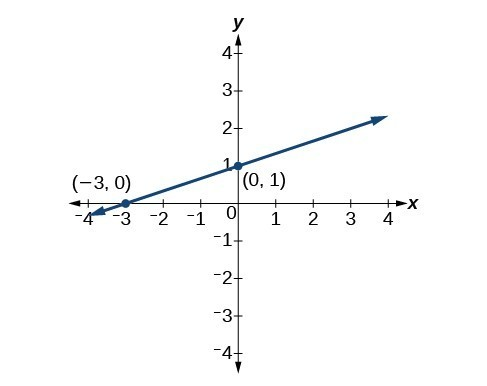Figure 7 ### Example 6: Writing Linear Equations Using Two Points Write the point-slope form of an equation of a line that passes through the points (5, 1) and (8, 7). Then rewrite it in the slope-intercept form. ### Try It Write the point-slope form of an equation of a line that passes through the points $\left(-1,3\right)$ and $\left(0,0\right)$. Then rewrite it in the slope-intercept form. ## Writing and Interpreting an Equation for a Linear Function Now that we have written equations for linear functions in both the slope-intercept form and the point-slope form, we can choose which method to use based on the information we are given. That information may be provided in the form of a graph, a point and a slope, two points, and so on. Look at the graph of the function f in Figure 8.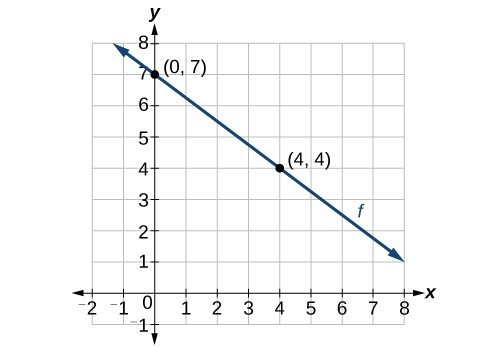Figure 8 We are not given the slope of the line, but we can choose any two points on the line to find the slope. Let’s choose (0, 7) and (4, 4). We can use these points to calculate the slope. \begin{align} m&=\frac{{y}_{2}-{y}_{1}}{{x}_{2}-{x}_{1}} \\ &=\frac{4 - 7}{4 - 0} \\ &=-\frac{3}{4} \end{align}  Now we can substitute the slope and the coordinates of one of the points into the point-slope form. $\begin{gathered}y-{y}_{1}=m\left(x-{x}_{1}\right) \\ y - 4=-\frac{3}{4}\left(x - 4\right) \end{gathered}$  If we want to rewrite the equation in the slope-intercept form, we would find $\begin{gathered}y - 4=-\frac{3}{4}\left(x - 4\right)\hfill \\ y - 4=-\frac{3}{4}x+3\hfill \\ \text{ }y=-\frac{3}{4}x+7\hfill \end{gathered}$Figure 9 If we wanted to find the slope-intercept form without first writing the point-slope form, we could have recognized that the line crosses the y-axis when the output value is 7. Therefore, b = 7. We now have the initial value b and the slope m so we can substitute m and b into the slope-intercept form of a line. So the function is $f(xt)=-\frac{3}{4}x+7$, and the linear equation would be $y=-\frac{3}{4}x+7$. ### How To: Given the graph of a linear function, write an equation to represent the function. 1. Identify two points on the line. 2. Use the two points to calculate the slope. 3. Determine where the line crosses the y-axis to identify the y-intercept by visual inspection. 4. Substitute the slope and y-intercept into the slope-intercept form of a line equation. ### Example 7: Writing an Equation for a Linear Function Write an equation for a linear function given a graph of f shown in Figure 10.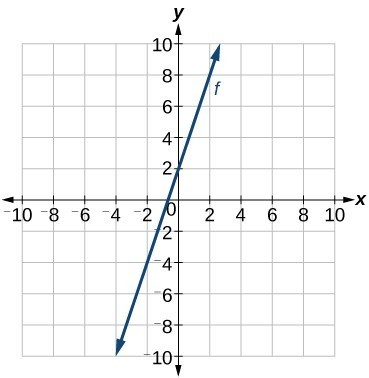Figure 10 ### Example 8: Writing an Equation for a Linear Function Given Two Points If f is a linear function, with $f\left(3\right)=-2$ , and $f\left(8\right)=1$, find an equation for the function in slope-intercept form. ### Try It If $f\left(x\right)$ is a linear function, with $f\left(2\right)=-11$, and $f\left(4\right)=-25$, find an equation for the function in slope-intercept form. ### Try it ## Graphing Linear Functions As we have seen, the graph of a linear function is a straight line. We were also able to see the points of the function as well as the initial value from a graph. By graphing two functions, then, we can more easily compare their characteristics. There are three basic methods of graphing linear functions. The first is by plotting points and then drawing a line through the points. The second is by using the y-intercept and slope. And the third is by using transformations of the identity function $f(x)=x$. ## Graphing a Function by Plotting Points To find points of a function, we can choose input values, evaluate the function at these input values, and calculate output values. The input values and corresponding output values form coordinate pairs. We then plot the coordinate pairs on a grid. In general, we should evaluate the function at a minimum of two inputs in order to find at least two points on the graph. For example, given the function, $f(x)=2x$, we might use the input values 1 and 2. Evaluating the function for an input value of 1 yields an output value of 2, which is represented by the point (1, 2). Evaluating the function for an input value of 2 yields an output value of 4, which is represented by the point (2, 4). Choosing three points is often advisable because if all three points do not fall on the same line, we know we made an error. ### How To: Given a linear function, graph by plotting points. 1. Choose a minimum of two input values. 2. Evaluate the function at each input value. 3. Use the resulting output values to identify coordinate pairs. 4. Plot the coordinate pairs on a grid. 5. Draw a line through the points. ### Example 9: Graphing by Plotting Points Graph $f\left(x\right)=-\frac{2}{3}x+5$ by plotting points. ### Try It Graph $f\left(x\right)=-\frac{3}{4}x+6$ by plotting points. ## Graphing a Linear Function Using y-intercept and Slope Another way to graph linear functions is by using specific characteristics of the function rather than plotting points. The first characteristic is its y-intercept, which is the point at which the input value is zero. To find the y-intercept, we can set x = 0 in the equation. The other characteristic of the linear function is its slope m, which is a measure of its steepness. Recall that the slope is the rate of change of the function. The slope of a function is equal to the ratio of the change in outputs to the change in inputs. Another way to think about the slope is by dividing the vertical difference, or rise, by the horizontal difference, or run. We encountered both the y-intercept and the slope in Linear Functions. Let’s consider the following function. $f\left(x\right)=\frac{1}{2}x+1$ The slope is $\frac{1}{2}$. Because the slope is positive, we know the graph will slant upward from left to right. The y-intercept is the point on the graph when = 0. The graph crosses the y-axis at (0, 1). Now we know the slope and the y-intercept. We can begin graphing by plotting the point (0,1) We know that the slope is rise over run, $m=\frac{\text{rise}}{\text{run}}$. From our example, we have $m=\frac{1}{2}$, which means that the rise is 1 and the run is 2. So starting from our y-intercept (0, 1), we can rise 1 and then run 2, or run 2 and then rise 1. We repeat until we have a few points, and then we draw a line through the points as shown in Figure 14.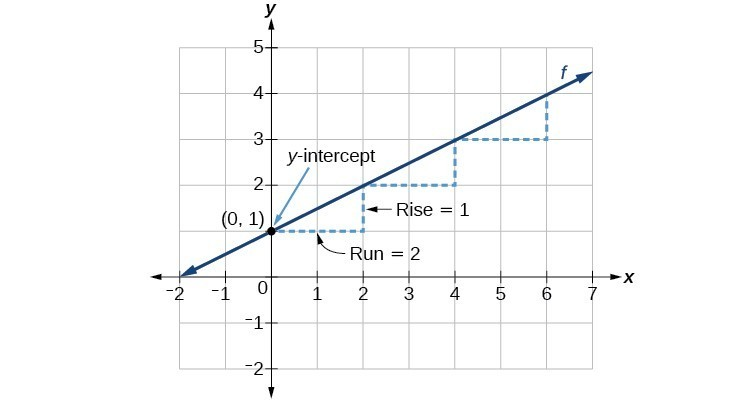Figure 14 ### A General Note: Graphical Interpretation of a Linear Function In the equation $f\left(x\right)=mx+b$ • b is the y-intercept of the graph and indicates the point (0, b) at which the graph crosses the y-axis. • m is the slope of the line and indicates the vertical displacement (rise) and horizontal displacement (run) between each successive pair of points. Recall the formula for the slope: $m=\frac{\text{change in output (rise)}}{\text{change in input (run)}}=\frac{\Delta y}{\Delta x}=\frac{{y}_{2}-{y}_{1}}{{x}_{2}-{x}_{1}}$ ### Q & A Do all linear functions have y-intercepts? Yes. All linear functions cross the y-axis and therefore have y-intercepts. (Note: A vertical line parallel to the y-axis does not have a y-intercept, but it is not a function.) ### How To: Given the equation for a linear function, graph the function using the y-intercept and slope. 1. Evaluate the function at an input value of zero to find the y-intercept. 2. Identify the slope as the rate of change of the input value. 3. Plot the point represented by the y-intercept. 4. Use $\frac{\text{rise}}{\text{run}}$ to determine at least two more points on the line. 5. Sketch the line that passes through the points. ### Example 10: Graphing by Using the y-intercept and Slope Graph $f\left(x\right)=-\frac{2}{3}x+5$ using the y-intercept and slope. ### Try It Find a point on the graph we drew in Example 10 that has a negative x-value. ### Try It ## Graphing a Linear Function Using Transformations Another option for graphing is to use transformations of the identity function $f(x)=x$ . A function may be transformed by a shift up, down, left, or right. A function may also be transformed using a reflection, stretch, or compression. ### Vertical Stretch or Compression In the equation $f(x)=mx$, the m is acting as the vertical stretch or compression of the identity function. When m is negative, there is also a vertical reflection of the graph. Notice in Figure 16 that multiplying the equation of $f(x)=x$ by m stretches the graph of f by a factor of m units if > 1 and compresses the graph of f by a factor of m units if 0 < < 1. This means the larger the absolute value of m, the steeper the slope.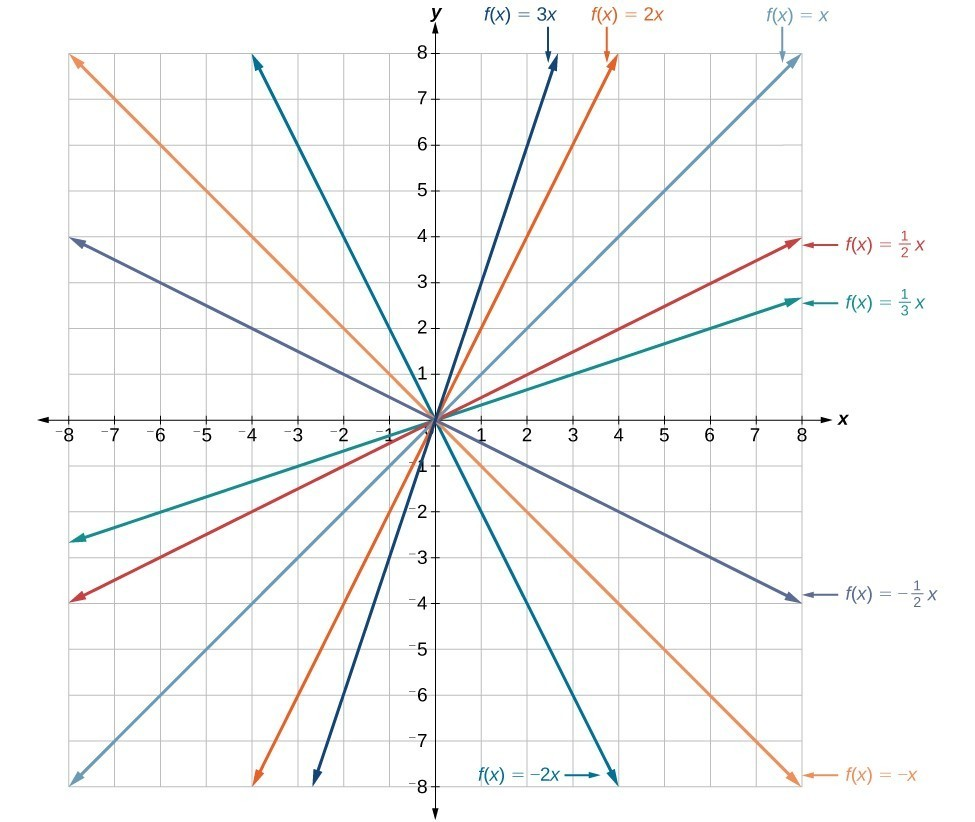Figure 16. Vertical stretches and compressions and reflections on the function $f\left(x\right)=x$. ### Vertical Shift In $f\left(x\right)=mx+b$, the b acts as the vertical shift, moving the graph up and down without affecting the slope of the line. Notice in Figure 17 that adding a value of b to the equation of $f\left(x\right)=x$ shifts the graph of f a total of b units up if b is positive and |b| units down if b is negative.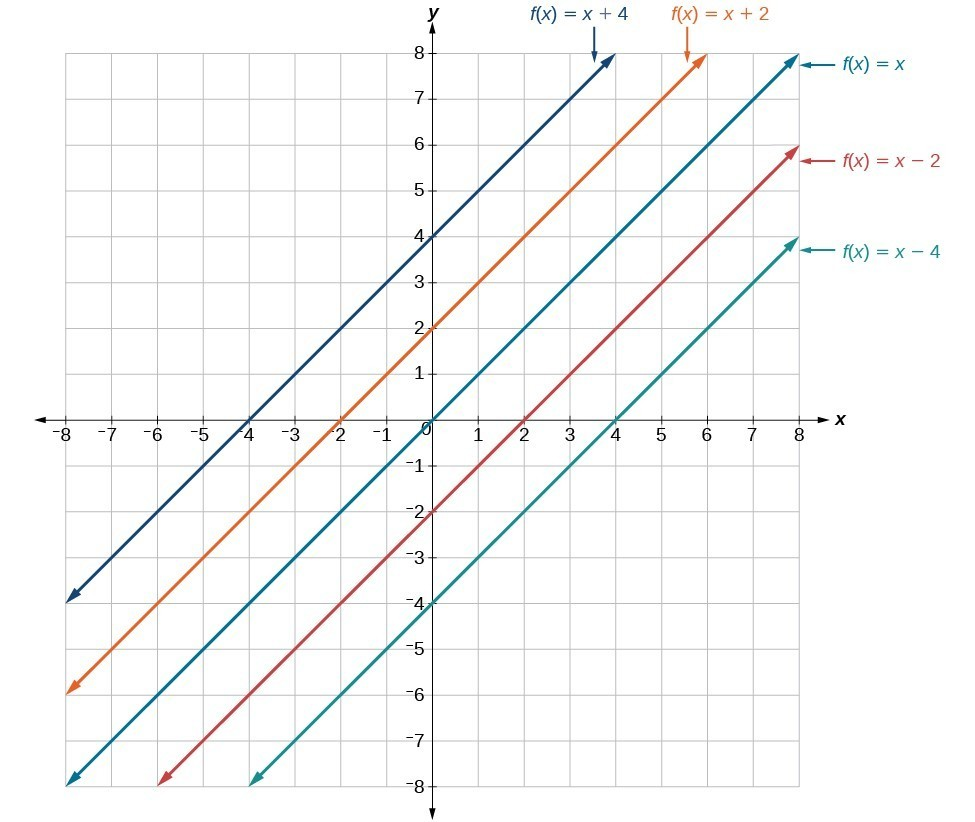Figure 17. This graph illustrates vertical shifts of the function $f(x)=x$. Using vertical stretches or compressions along with vertical shifts is another way to look at identifying different types of linear functions. Although this may not be the easiest way to graph this type of function, it is still important to practice each method. ### How To: Given the equation of a linear function, use transformations to graph the linear function in the form $f\left(x\right)=mx+b$. 1. Graph $f\left(x\right)=x$. 2. Vertically stretch or compress the graph by a factor m. 3. Shift the graph up or down b units. ### Example 11: Graphing by Using Transformations Graph $f\left(x\right)=\frac{1}{2}x - 3$ using transformations. ### Try It Graph $f\left(x\right)=4+2x$, using transformations. ### Q & A In Example 3, could we have sketched the graph by reversing the order of the transformations? No. The order of the transformations follows the order of operations. When the function is evaluated at a given input, the corresponding output is calculated by following the order of operations. This is why we performed the compression first. For example, following the order: Let the input be 2. \begin{align}f(2)&=\frac{1}{2}(2)-3 \\ &=1-3 \\ &=-2 \end{align} ## Writing the Equation for a Function from the Graph of a Line Earlier, we wrote the equation for a linear function from a graph. Now we can extend what we know about graphing linear functions to analyze graphs a little more closely. Begin by taking a look at Figure 21. We can see right away that the graph crosses the y-axis at the point (0, 4) so this is the y-intercept.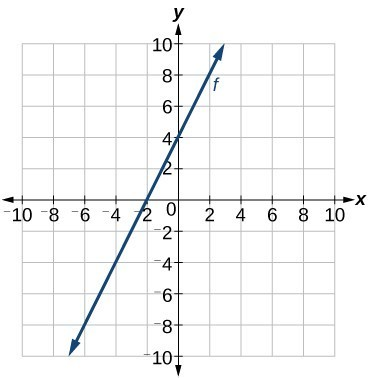Figure 21 Then we can calculate the slope by finding the rise and run. We can choose any two points, but let’s look at the point (–2, 0). To get from this point to the y-intercept, we must move up 4 units (rise) and to the right 2 units (run). So the slope must be $m=\frac{\text{rise}}{\text{run}}=\frac{4}{2}=2$ Substituting the slope and y-intercept into the slope-intercept form of a line gives $y=2x+4$ ### How To: Given a graph of linear function, find the equation to describe the function. 1. Identify the y-intercept of an equation. 2. Choose two points to determine the slope. 3. Substitute the y-intercept and slope into the slope-intercept form of a line. ### Example 12: Matching Linear Functions to Their Graphs Match each equation of the linear functions with one of the lines in Figure 22. 1. $f\left(x\right)=2x+3$ 2. $g\left(x\right)=2x - 3$ 3. $h\left(x\right)=-2x+3$ 4. $j\left(x\right)=\frac{1}{2}x+3$ ## Finding the x-intercept of a Line So far, we have been finding the y-intercepts of a function: the point at which the graph of the function crosses the y-axis. A function may also have an x-intercept, which is the x-coordinate of the point where the graph of the function crosses the x-axis. In other words, it is the input value when the output value is zero. To find the x-intercept, set a function f(x) equal to zero and solve for the value of x. For example, consider the function shown. $f\left(x\right)=3x - 6$ Set the function equal to 0 and solve for x. \begin{align}&0=3x - 6 \\ &6=3x \\ &2=x \\ &x=2 \end{align} The graph of the function crosses the x-axis at the point (2, 0). ### Q & A Do all linear functions have x-intercepts? No. However, linear functions of the form = c, where c is a nonzero real number are the only examples of linear functions with no x-intercept. For example, = 5 is a horizontal line 5 units above the x-axis. This function has no x-intercepts.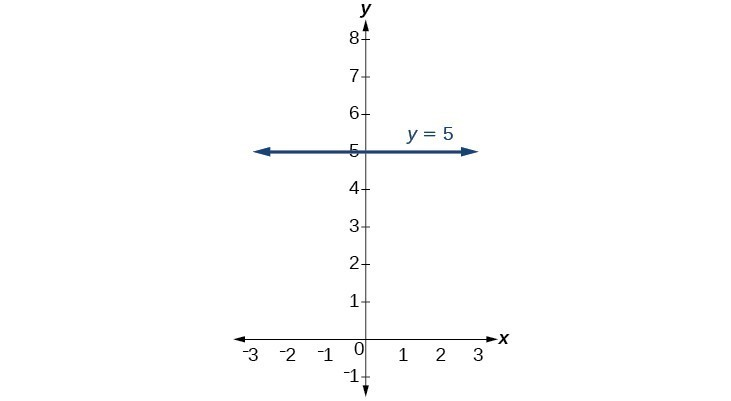Figure 24 ### A General Note: x-intercept The x-intercept of the function is the point where the graph crosses the x-axis. Points on the x-axis have the form (x,0) so we can find x-intercepts by setting f(x) = 0. For a linear function, we solve the equation mx + = 0 ### Example 13: Finding an x-intercept Find the x-intercept of $f\left(x\right)=\frac{1}{2}x - 3$. ### Try It Find the x-intercept of $f\left(x\right)=\frac{1}{4}x - 4$. ## Describing Horizontal and Vertical Lines There are two special cases of lines on a graph—horizontal and vertical lines. A horizontal line indicates a constant output, or y-value. In Figure 26, we see that the output has a value of 2 for every input value. The change in outputs between any two points, therefore, is 0. In the slope formula, the numerator is 0, so the slope is 0. If we use = 0 in the equation $f(x)=mx+b$, the equation simplifies to $f(x)=b$. In other words, the value of the function is a constant. This graph represents the function $f(x)=2$.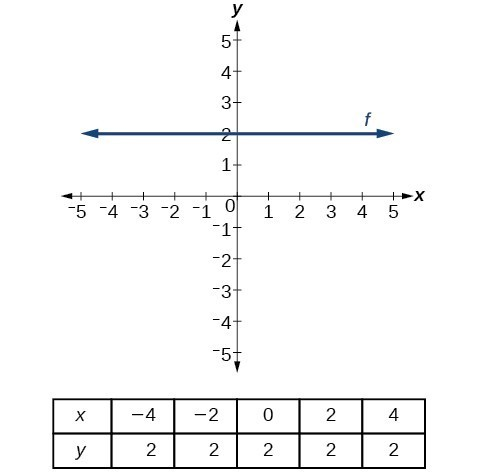Figure 26. A horizontal line representing the function $f(x)=2$.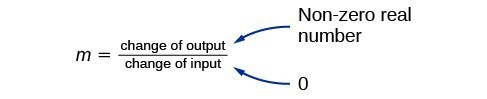Figure 27 A vertical line indicates a constant input, or x-value. We can see that the input value for every point on the line is 2, but the output value varies. Because this input value is mapped to more than one output value, a vertical line does not represent a function. Notice that between any two points, the change in the input values is zero. In the slope formula, the denominator will be zero, so the slope of a vertical line is undefined. Notice that a vertical line, such as the one in Figure 28, has an x-intercept, but no y-intercept unless it’s the line x = 0. This graph represents the line x = 2.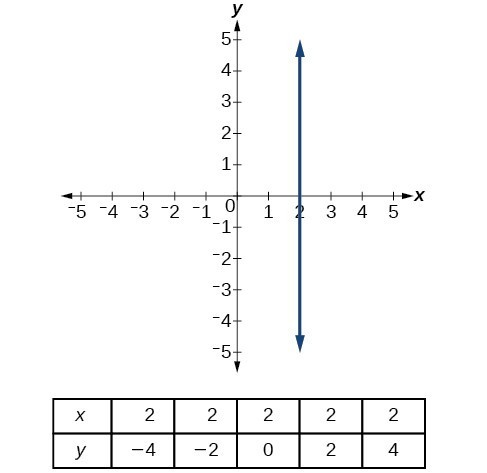Figure 28. The vertical line, = 2, which does not represent a function. ### A General Note: Horizontal and Vertical Lines Lines can be horizontal or vertical. A horizontal line is a line defined by an equation in the form $f(x)=b$. A vertical line is a line defined by an equation in the form $x=a$. ### Example 14: Writing the Equation of a Horizontal Line Write the equation of the line graphed in Figure 29.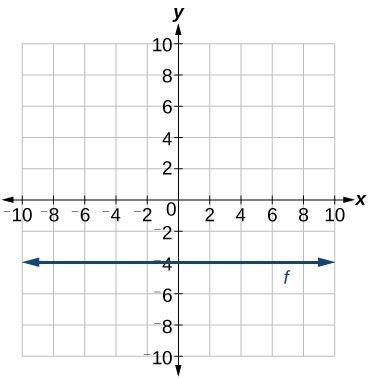Figure 29 ### Example 15: Writing the Equation of a Vertical Line Write the equation of the line graphed in Figure 30.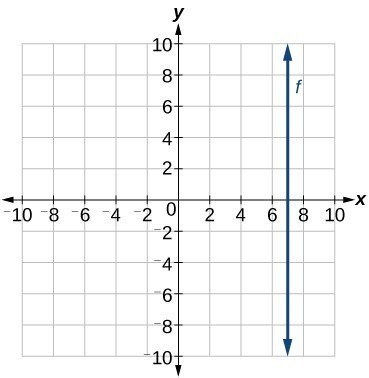Figure 30 ## Key Equations  slope-intercept form of a line $f\left(x\right)=mx+b$ slope $m=\frac{\text{change in output (rise)}}{\text{change in input (run)}}=\frac{\Delta y}{\Delta x}=\frac{{y}_{2}-{y}_{1}}{{x}_{2}-{x}_{1}}$ point-slope form of a line $y-{y}_{1}=m\left(x-{x}_{1}\right)$ ## Key Concepts • The ordered pairs given by a linear function represent points on a line. • Linear functions can be represented in words, function notation, tabular form, and graphical form. • The rate of change of a linear function is also known as the slope. • An equation in the slope-intercept form of a line includes the slope and the initial value of the function. • The initial value, or y-intercept, is the output value when the input of a linear function is zero. It is the y-value of the point at which the line crosses the y-axis. • An increasing linear function results in a graph that slants upward from left to right and has a positive slope. • A decreasing linear function results in a graph that slants downward from left to right and has a negative slope. • A constant linear function results in a graph that is a horizontal line. • Analyzing the slope within the context of a problem indicates whether a linear function is increasing, decreasing, or constant. • The slope of a linear function can be calculated by dividing the difference between y-values by the difference in corresponding x-values of any two points on the line. • The slope and initial value can be determined given a graph or any two points on the line. • One type of function notation is the slope-intercept form of an equation. • The point-slope form is useful for finding a linear equation when given the slope of a line and one point. • The point-slope form is also convenient for finding a linear equation when given two points through which a line passes. • The equation for a linear function can be written if the slope m and initial value are known. • A linear function can be used to solve real-world problems. • A linear function can be written from tabular form. • Linear functions may be graphed by plotting points or by using the y-intercept and slope. • Graphs of linear functions may be transformed by using shifts up, down, left, or right, as well as through stretches, compressions, and reflections. • The y-intercept and slope of a line may be used to write the equation of a line. • The x-intercept is the point at which the graph of a linear function crosses the x-axis. • Horizontal lines are written in the form, f(x) = b. • Vertical lines are written in the form, = b. ## Glossary decreasing linear function a function with a negative slope: If $f\left(x\right)=mx+b, \text{then } m<0$. horizontal line a line defined by $f(x)=b$, where b is a real number. The slope of a horizontal line is 0. increasing linear function a function with a positive slope: If $f\left(x\right)=mx+b, \text{then } m>0$. linear function a function with a constant rate of change that is a polynomial of degree 1, and whose graph is a straight line point-slope form the equation for a line that represents a linear function of the form $y-{y}_{1}=m\left(x-{x}_{1}\right)$ slope the ratio of the change in output values to the change in input values; a measure of the steepness of a line slope-intercept form the equation for a line that represents a linear function in the form $f\left(x\right)=mx+b$ vertical line a line defined by x = a, where a is a real number. The slope of a vertical line is undefined. x-intercept the point on the graph of a linear function when the output value is 0; the point at which the graph crosses the horizontal axis y-intercept the value of a function when the input value is zero; also known as initial value ## Section 1.4 Homework Exercises 1. Terry is skiing down a steep hill. Terry’s elevation, E(t), in feet after t seconds is given by $E\left(t\right)=3000-70t$. Write a complete sentence describing Terry’s starting elevation and how it is changing over time. 2. Maria is climbing a mountain. Maria’s elevation, E(t), in feet after t minutes is given by $E\left(t\right)=1200+40t$. Write a complete sentence describing Maria’s starting elevation and how it is changing over time. 3. Jessica is walking home from a friend’s house. After 2 minutes she is 1.4 miles from home. Twelve minutes after leaving, she is 0.9 miles from home. What is her rate in miles per hour? 4. Sonya is currently 10 miles from home and is walking farther away at 2 miles per hour. Write an equation for her distance from home t hours from now. 5. A boat is 100 miles away from the marina, sailing directly toward it at 10 miles per hour. Write an equation for the distance of the boat from the marina after t hours. 6. Timmy goes to the fair with40. Each ride costs \$2. How much money will he have left after riding $n$ rides?

For the following exercises, determine whether the equation of the curve can be written as a linear function.

7. $y=\frac{1}{4}x+6$

8. $y=3x - 5$

9. $y=3{x}^{2}-2$

10. $3x+5y=15$

11. $3{x}^{2}+5y=15$

12. $3x+5{y}^{2}=15$

13. $-2{x}^{2}+3{y}^{2}=6$

14. $-\frac{x - 3}{5}=2y$

For the following exercises, determine whether each function is increasing or decreasing.

15. $f\left(x\right)=4x+3$

16. $g\left(x\right)=5x+6$

17. $a\left(x\right)=5 - 2x$

18. $b\left(x\right)=8 - 3x$

19. $h\left(x\right)=-2x+4$

20. $k\left(x\right)=-4x+1$

21. $j\left(x\right)=\frac{1}{2}x - 3$

22. $p\left(x\right)=\frac{1}{4}x - 5$

23. $n\left(x\right)=-\frac{1}{3}x - 2$

24. $m\left(x\right)=-\frac{3}{8}x+3$

For the following exercises, find the slope of the line that passes through the two given points.

25. $\left(2,\text{ }4\right)$ and $\left(4,\text{ 10}\right)$

26. $\left(1,\text{ 5}\right)$ and $\left(4,\text{ 11}\right)$

27. $\left(-1,\text{4}\right)$ and $\left(5,\text{2}\right)$

28. $\left(8,-2\right)$ and $\left(4,6\right)$

29. $\left(6,\text{ }11\right)$ and $\left(-4, 3\right)$

For the following exercises, given each set of information, find a linear equation satisfying the conditions, if possible.

30. $f\left(-5\right)=-4$, and $f\left(5\right)=2$

31. $f\left(-1\right)=4$ and $f\left(5\right)=1$

32. $\left(2,4\right)$ and $\left(4,10\right)$

33. Passes through $\left(1,5\right)$ and $\left(4,11\right)$

34. Passes through $\left(-1,\text{ 4}\right)$ and $\left(5,\text{ 2}\right)$

35. Passes through $\left(-2,\text{ 8}\right)$ and $\left(4,\text{ 6}\right)$

36. x intercept at $\left(-2,\text{ 0}\right)$ and y intercept at $\left(0,-3\right)$

37. x intercept at $\left(-5,\text{ 0}\right)$ and y intercept at $\left(0,\text{ 4}\right)$

For the following exercises, find the slope of the lines graphed.

38.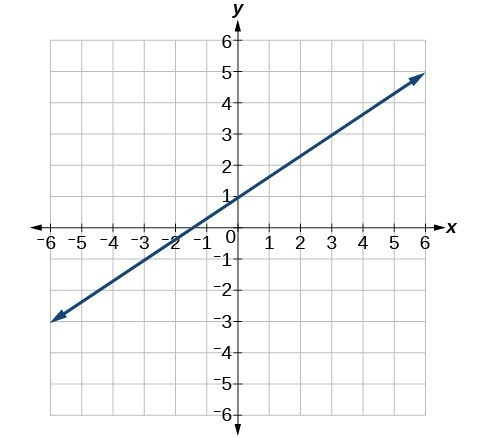39.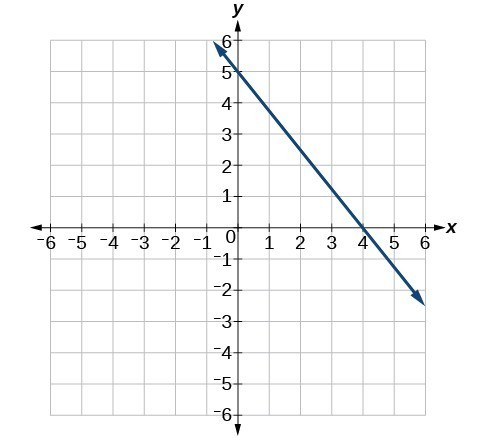40.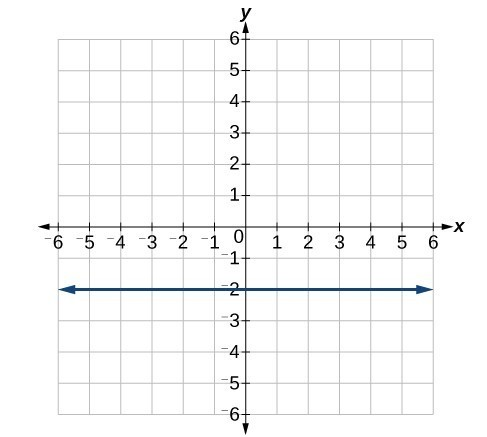For the following exercises, write an equation for the lines graphed.

41.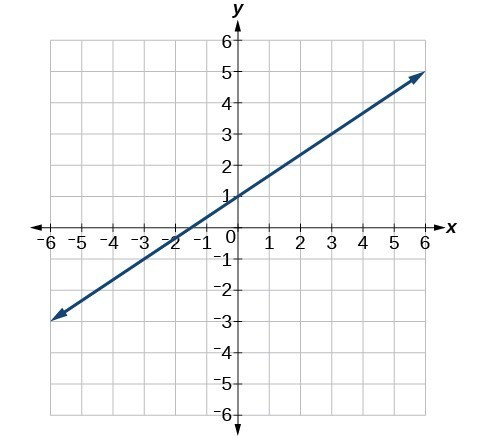42.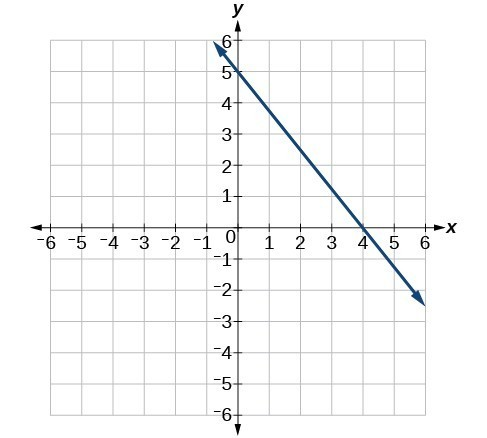43.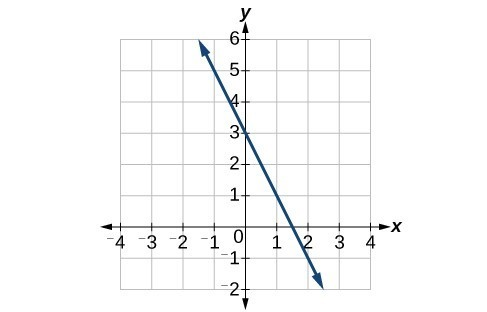44.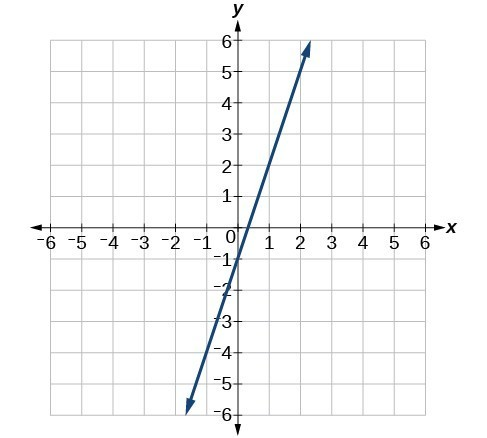45.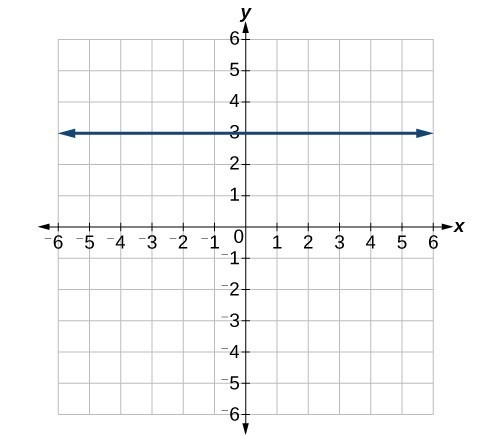46.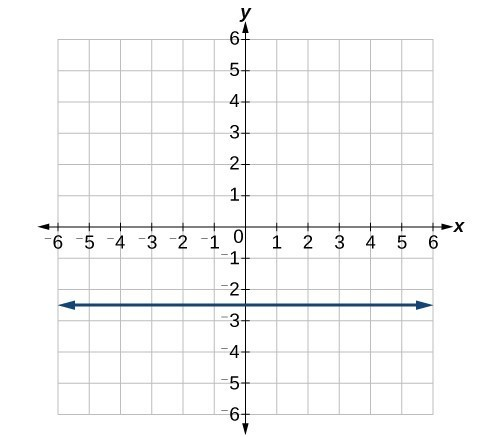For the following exercises, which of the tables could represent a linear function? For each that could be linear, find a linear equation that models the data.

47.

 x 0 5 10 15 g(x) 5 –10 –25 –40

48.

 x 0 5 10 15 h(x) 5 30 105 230

49.

 x 0 5 10 15 f(x) –5 20 45 70

50.

 x 5 10 20 25 k(x) 28 13 58 73

51.

 x 0 2 4 6 g(x) 6 –19 –44 –69

52.

 x 2 4 6 8 f(x) –4 16 36 56

53.

 x 2 4 6 8 f(x) –4 16 36 56

54.

 x 0 2 6 8 k(x) 6 31 106 231

55. Find the value of x if a linear function goes through the following points and has the following slope: $\left(x,2\right),\left(-4,6\right),m=3$

56. Find the value of y if a linear function goes through the following points and has the following slope: $\left(10,y\right),\left(25,100\right),m=-5$

57. Find the equation of the line that passes through the following points: $\left(a,\text{ }b\right)$ and $\left(a,\text{ }b+1\right)$

58. Find the equation of the line that passes through the following points: $\left(2a,\text{ }b\right)$ and $\left(a,\text{ }b+1\right)$

59. Find the equation of the line that passes through the following points: $\left(a,\text{ }0\right)$ and $\left(c,\text{ }d\right)$

For the following exercises, match the given linear equation with its graph.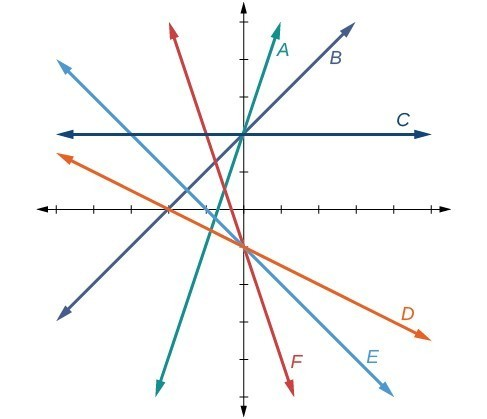60. $f\left(x\right)=-x - 1$

61. $f\left(x\right)=-2x - 1$

62. $f\left(x\right)=-\frac{1}{2}x - 1$

63. $f\left(x\right)=2$

64. $f\left(x\right)=2+x$

65. $f\left(x\right)=3x+2$

For the following exercises, sketch a line with the given features.

66. An x-intercept of $\left(-\text{4},\text{ 0}\right)$ and y-intercept of $\left(0,\text{ -2}\right)$

67. An x-intercept of $\left(-\text{2},\text{ 0}\right)$ and y-intercept of $\left(0,\text{ 4}\right)$

68. A y-intercept of $\left(0,\text{ 7}\right)$ and slope $-\frac{3}{2}$

69. A y-intercept of $\left(0,\text{ 3}\right)$ and slope $\frac{2}{5}$

70. Passing through the points $\left(-\text{6},\text{ -2}\right)$ and $\left(\text{6},\text{ -6}\right)$

71. Passing through the points $\left(-\text{3},\text{ -4}\right)$ and $\left(\text{3},\text{ 0}\right)$

For the following exercises, sketch the graph of each equation.

72. $f\left(x\right)=-2x - 1$

73. $g\left(x\right)=-3x+2$

74. $h\left(x\right)=\frac{1}{3}x+2$

75. $k\left(x\right)=\frac{2}{3}x - 3$

76. $f\left(t\right)=3+2t$

77. $p\left(t\right)=-2+3t$

78. $x=3$

79. $x=-2$

80. $r\left(x\right)=4$

81. $q\left(x\right)=3$

82. $4x=-9y+36$

83. $\frac{x}{3}-\frac{y}{4}=1$

84. $3x - 5y=15$

85. $3x=15$

86. $3y=12$

87. If $g\left(x\right)$ is the transformation of $f\left(x\right)=x$ after a vertical compression by $\frac{3}{4}$, a shift right by 2, and a shift down by 4

a. Write an equation for $g\left(x\right)$.

b. What is the slope of this line?

c. Find the y-intercept of this line.

88. If $g\left(x\right)$ is the transformation of $f\left(x\right)=x$ after a vertical compression by $\frac{1}{3}$, a shift left by 1, and a shift up by 3

a. Write an equation for $g\left(x\right)$.

b. What is the slope of this line?

c. Find the y-intercept of this line.

For the following exercises, write the equation of the line shown in the graph.

89.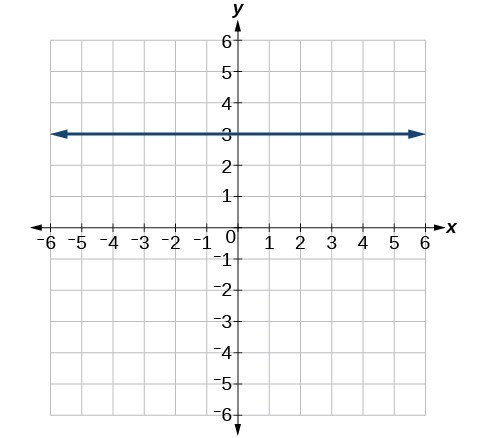90.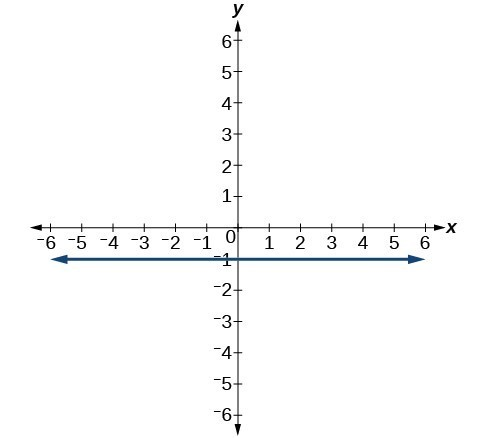91.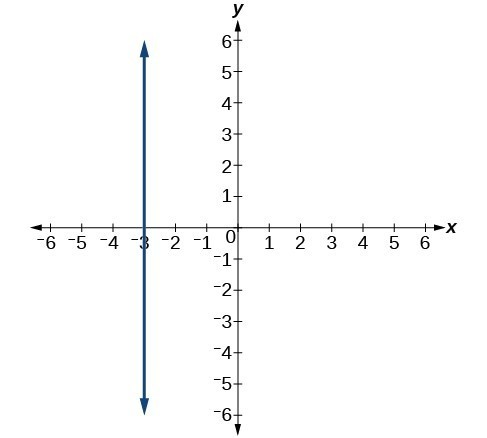92.